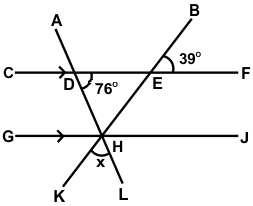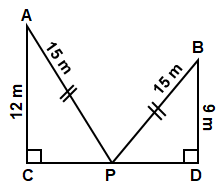# CREST Mathematics Olympiad Class 7 Sample Papers

## Syllabus:

Section 1: Integers, Fractions and Decimals, Exponents and Powers, Algebraic Expressions, Simple Linear Equations, Lines and Angles, Comparing Quantities, The Triangle and its Properties, Symmetry, Congruence of Triangles, Rational Numbers, Perimeter and Area, Data Handling, Visualizing Solid Shapes, Practical Geometry.

Achievers Section: Higher Order Thinking Questions - Syllabus as per Section 1

 Q.1 An article is listed at \$900 and two successive discounts of 8% and 8% are given on it. How much would the seller gain or lose, if he gives a single discount of 16%, instead of two discounts?
 Q.2 A submarine submerges at the rate of 5 m per minute. If it descends from 20 m above the sea level, how long will it take to reach 250 m below the sea level:
 Q.3 40% of 60% of 3/5th of a number is 504. What is 25% of 2/5th of that number?
 Q.4 A class has girls and boys in the ratio 4:5, Among the girls, the ratio of mathematics to physics students is 3:1. If the ratio of mathematics and physics students in the entire class is 3:2, then what percentage of class comprises girls studying mathematics?
 Q.5 The difference between a two-digit number and the number formed by reversing the digits is always divisible by ______.

Q.6

Frame the formula from the following table and make x as the subject of the formula:

 x 3 5 7 9 11 y 5 9 13 17 21

 Q.7 Find the value of ∠x:Q.8 LM is a straight line and O is a point on LM. Line ON is drawn not coinciding with OL or OM. If ∠MON is three times of ∠LON, then ∠MON is equal to:
 Q.9 Ross scored 61 out of 100 in English, 93 out of 100 in Maths and 56 out of 100 in Science. Find out his overall % of marks:
 Q.10 Find the length of CD:Sample PDF of CREST Mathematics Olympiad for Class 7:

Answers to Sample Questions from CREST Olympiads:

 Q.1 b Q.2 b Q.3 d Q.4 a Q.5 a Q.6 d Q.7 d Q.8 d Q.9 b Q.10 c

CREST Mathematics Olympiad is an internationally conducted exam for students of class 7. This examination requires dedicated and efficient preparation to score good marks and rank in the examination. One of the best ways to excel in preparation is to practice Maths Olympiad sample papers. The sample questions will help participating students get a close understanding of the types of questions generally asked in the CMO exam. To have a good grip on the subject students must definitely attempt at least 3 sample papers.

Practicing Maths Olympiad previous years’ question papers for class 7 gives students an actual examination feel. Participants can download for class 7 from this page. The sample paper is divided into various small sections that include multiple choice questions and covers each and every topic of the syllabus. This way students will develop a good problem-solving capability which will further help them during the examination. For additional Maths Olympiad questions, students can give 2 free practice tests.

Answers to Sample Questions from CREST Olympiads:

Q.1 : b | Q.2 : b | Q.3 : d | Q.4 : a | Q.5 : a | Q.6 : d | Q.7 : d | Q.8 : d | Q.9 : b | Q.10 : c

70%